# Question - how do you know that they are all 3-4-5 triangles?

• Module 2 Week 2 Day 5 Your Turn Mini-Question Part 3

In the question explanation, how do you know that they are all 3-4-5 triangles?

• @divinedolphin That's a good question! You can "angle chase" starting from the little triangle that is labeled with sides of \(3, 4,\) and \(5.\) Let's start by coloring one of its angles purple and the other angle yellow. The purple and yellow angles add up to \(90^{\circ}\) since all the angles in a triangle add up to \(180^{\circ}.\)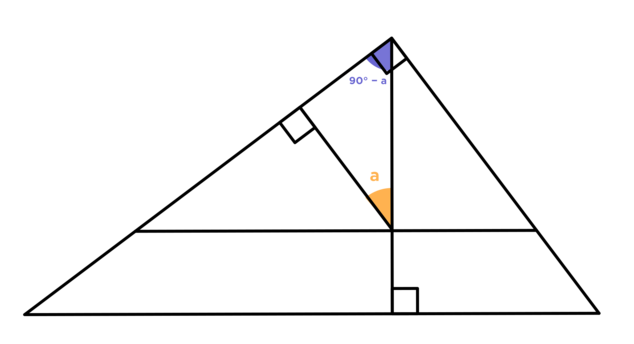Now, we can "walk" over to the angle that is adjacent to the purple angle. Since it makes a right angle with the purple angle, we know that it is complementary to the purple angle, and so is equal to the yellow angle.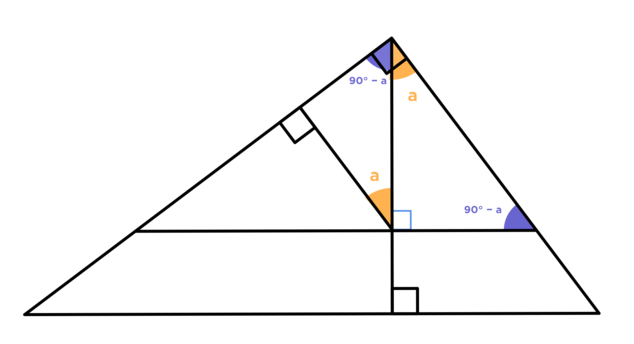Now we know that the other angle of this triangle adds up to \(90^{\circ}\) along with the yellow angle, so it is complementary to the yellow angle and so is equal to the purple angle. Since the two horizontal lines are parallel, the angle in the bottom right is also equal to the purple angle. Now there is another yellow angle on the left, because it forms a triangle with the purple angle that we just found: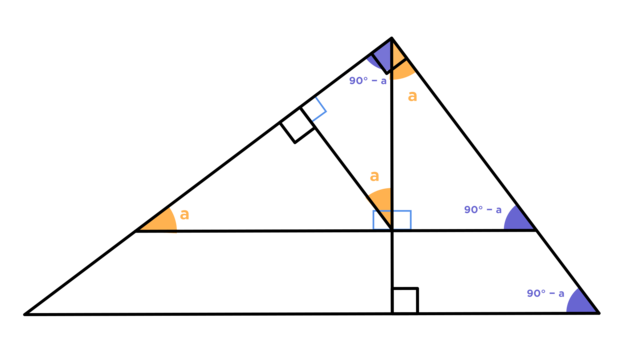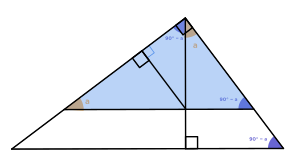Since this yellow angle is part of a right triangle, the other angle in it is complementary to the yellow angle and so is equal to the purple angle: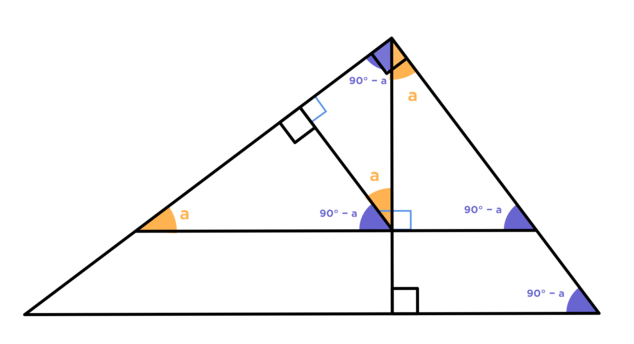Finally, looking at the entire large triangle, we see that the very bottom left angle must be equal to the yellow angle, since it is complementary with the purple angle on the right. Another way is to use the fact that the two horizontal lines give equal angles \(\angle a.\)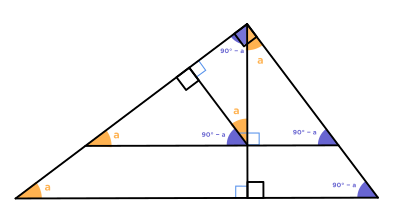So, we have yellow and purple angles throughout the entire diagram, which means all of these triangles are similar to the \(3-4-5\)-right triangle!